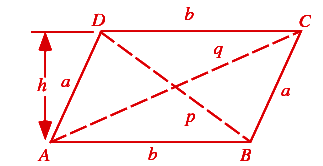# Height of a Parallelogram Formula

Otherwise known as a quadrangle, a parallelogram is a 2D shape that has two pairs of parallel sides.
The base and height of the parallelogram are perpendicular.  The diagonals of a parallelogram bisect each other. The formula for the height of the parallelogram is as follows:## Formula of Height of a Parallelogram

$\LARGE Height=\, \frac{Area}{Base}$

### Solved example:

Question. What will be the height of the parallelogram if the area  is 30 Sq inch and the base of a parallelogram is 6 inch, then find the height of a parallelogram ?

Solution.
The height of the parallelogram is

$$\begin{array}{l}Height=\, \frac{Area}{Base}\end{array}$$

a= 30,  b = 6

H =

$$\begin{array}{l}\frac{30}{6}\end{array}$$
= 5cm

Hence, the height is 5 cm.